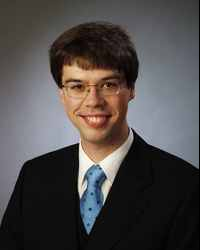Search:Contact:

Herr Ralf Meyer
Bunsenstr. 3-5
37073 Göttingen

Phone.: +49 551 39 27774
Fax.: +49 551 39 27181
E-Mail: ralf.meyer@mathematik.uni-goettingen.de
URL: http://www.uni-math.gwdg.de/rameyer/
Office hours: appointment by email

# Some more publications

## Bücher

• Funktionentheorie (Hermann Weyl).
Birkhäuser, ISBN 978-3-7643-8845-4

This is a transcription of a lecture course by Hermann Weyl from 1910–11, which I edited as a project for a LaTeX course.

A list of Errata was kindly provided to me by Horst Petereit.

• Local and Analytic Cyclic Homology.
European Mathematical Society Publishing House, EMS Tracts in Mathematics, vol. 3, August 2007, 368 pages.
ISBN 978-3-03719-039-5

Abstract: Periodic cyclic homology is a homology theory for non-commutative algebras that plays a similar role in non-commutative geometry as de Rham cohomology for smooth manifolds. While it produces good results for algebras of smooth or polynomial functions, it fails for bigger algebras such as most Banach algebras or ${C}^{*}$-algebras. Analytic and local cyclic homology are variants of periodic cyclic homology that work better for such algebras. In this book the author develops and compares these theories, emphasising their homological properties. This includes the excision theorem, invariance under passage to certain dense subalgebras, a Universal Coefficient Theorem that relates them to K-theory, and the Chern-Connes character for K-theory and K-homology.

The cyclic homology theories studied in this text require a good deal of functional analysis in bornological vector spaces, which is supplied in the first chapters. The focal points here are the relationship with inductive systems and the functional calculus in non-commutative bornological algebras.

The book is mainly intended for researchers and advanced graduate students interested in non-commutative geometry. Some chapters are more elementary and independent of the rest of the book, and will be of interest to researchers and students working in functional analysis and its applications.

• Topological and Bivariant K-Theory.
Joint work with Joachim Cuntz and Jonathan M. Rosenberg.
Birkhäuser, Oberwolfach Seminars, vol. 36, 2007, 262 pages.
ISBN: 978-3-7643-8398-5

Abstract: Topological K-theory is one of the most important invariants for noncommutative algebras equipped with a suitable topology or bornology. Bott periodicity, homotopy invariance, and various long exact sequences distinguish it from algebraic K-theory.

We describe a bivariant K-theory for bornological algebras, which provides a vast generalization of topological K-theory. In addition, we discuss other approaches to bivariant K-theories for operator algebras. As applications, we study K-theory of crossed products, the Baum-Connes assembly map, twisted K-theory with some of its applications, and some variants of the Atiyah-Singer Index Theorem.

## Weitere Arbeiten

• Homological algebra for Schwartz algebras. In: Symmetries in Algebra and Number Theory, Proceedings of the Göttingen-Jerusalem Conference (Göttingen, October 27-30, 2008), Universitätsverlag Göttingen, 2009, ISBN: 978-3-940344-96-0. Pages 99-114.

Abstract: Let G be a reductive group over a non-Archimedean local field. For two tempered smooth representations, it makes no difference for the Ext-groups whether we work in the category of tempered smooth representations of G or of all smooth representations of G. Similar results hold for certain discrete groups. We explain the basic ideas from functional analysis and geometric group theory that are needed to state this result correctly and prove it.

• Dimensional Regularization.
This is an elaboration of my lecture at the workshop ``Theory of Renormalization and Regularization'' in Hesselberg 2002.

Abstract:  We explain the mathematical theory needed for dimensional regularization, which is a way to regularize divergent integrals that is used in popular renormalization schemes of perturbative quantum field theory.

• The Mayer-Vietoris Sequence for Generalized Homology Theories.

Abstract: We give a simple proof that generalized homology theories on categories of algebras have a long exact Mayer-Vietoris sequence computing the homology of a pull-back.

• Cyclic cohomology theories and nilpotent extensions.
This is a slightly modified version of my dissertation, written at the Westfälische Wilhelms-Universität under the direction of Prof. Dr. Joachim Cuntz.

Abstract: We develop analytic cyclic cohomology for complete bornological algebras, a version of entire cyclic cohomology. The main results are a conceptual proof of excision for this theory and the construction of a bivariant Chern-Connes character for Fredholm modules without summability restrictions. The proof of excision applies also to periodic cyclic cohomology of complete bornological algebras or topological algebras (even of pro-algebras).

• Exercises and Solutions for a Course on Lie Groups and Lie Algebras.
These are the solutions to the exercises for the Fall 1997 Math 261A course at the University of California at Berkeley taught by Vera Serganova.

• Morita Equivalence in Algebra and Geometry.
This article has been prepared for the Spring 1997 Math 277 course at the University of California at Berkeley taught by Alan Weinstein.

Abstract: We study the notion of Morita equivalence in various categories. We start with Morita equivalence and Morita duality in pure algebra. Then we consider strong Morita equivalence for ${C}^{*}$-algebras and Morita equivalence for W*-algebras. Finally, we examine the corresponding notions for groupoids (with structure) and Poisson manifolds.

• The Carathéodory Distance and Linear Functionals.